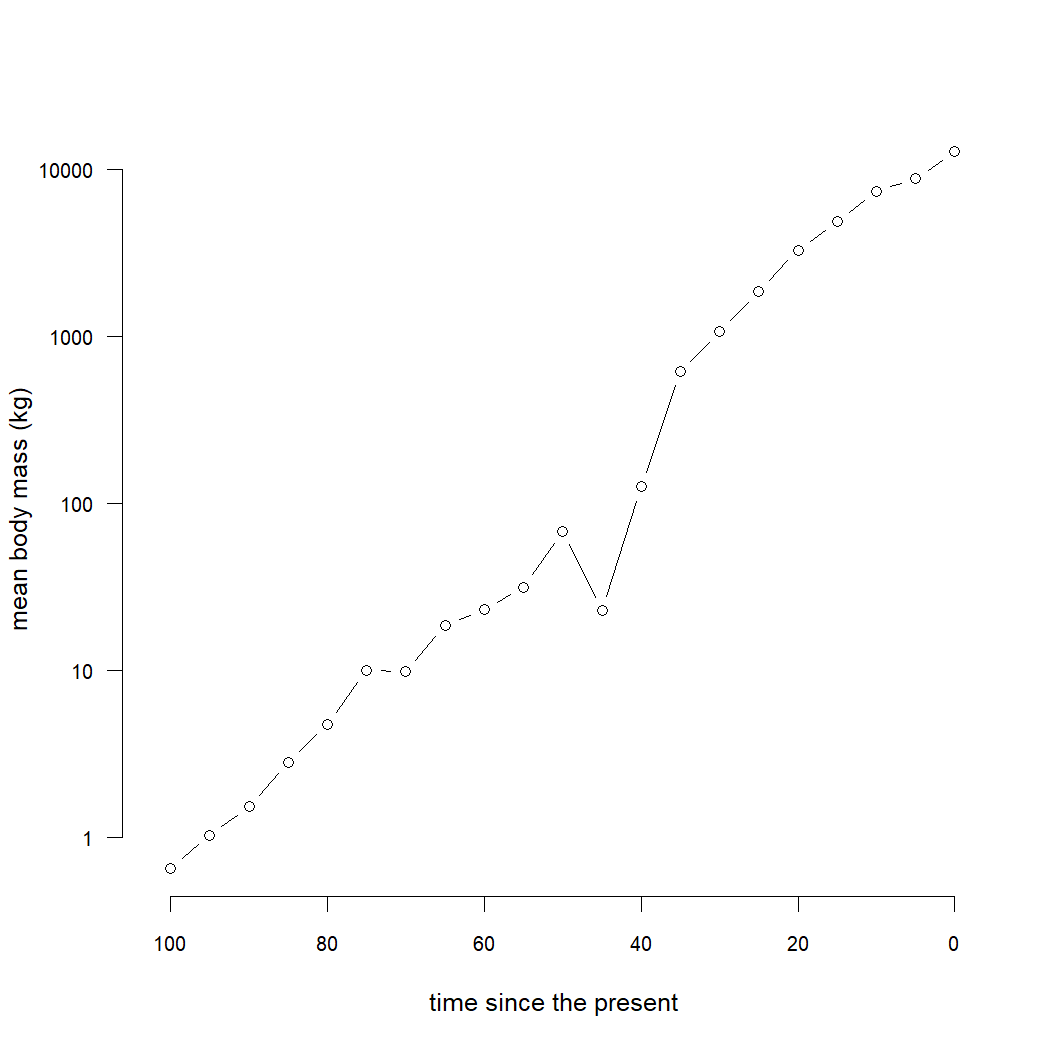## Saturday, November 13, 2021

### Graphing the maximum (or mean) estimated (or observed) value of a continuous trait through time on a phylogenetic tree

Yesterday a friend & collaborator asked me how to compute the observed or reconstructed maximum value of a continuous character trait through time on a phylogeny

The idea here is to imagine cutting slices through our phylogenetic tree at a set of pre-determined time points, estimate the ancestral state along each edge bisected by this slice, and then find the maximum value of this set of point estimates.

In his case, he was working with a phylogeny consisting of both extinct and extant lineages. This is good, because it should give us a lot more confidence in our set of reconstructed values in each time slice. (Though we could also do it for an extant-only tree.)

To start off, I'll simulate a phylogenetic tree of extant and extinct species, and then I'll simulate a single character on that tree using Brownian motion. For fun, let's just imagine that the trait which is evolving is log(body mass) in log(kg).

``````set.seed(31)
``````
``````## load packages
library(phytools)
## simulate tree & data
N<-20
t<-102
tree<-pbtree(n=N,b=1,d=0.6,scale=t)
while(length(getExtant(tree))<20)
tree<-pbtree(n=N,b=1,d=0.6,scale=t)
x<-fastBM(tree,sig2=0.1)
``````

To get a sense of our data, let's graph a projection of our tree into traitspace using the phytools `phenogram` function as follows.

``````a<-fastAnc(tree,x)
options(scipen=6)
phenogram(tree,exp(c(a,x)),ftype="off",log="y",las=1,
cex.axis=0.8,ylab="body mass (kg)")
``````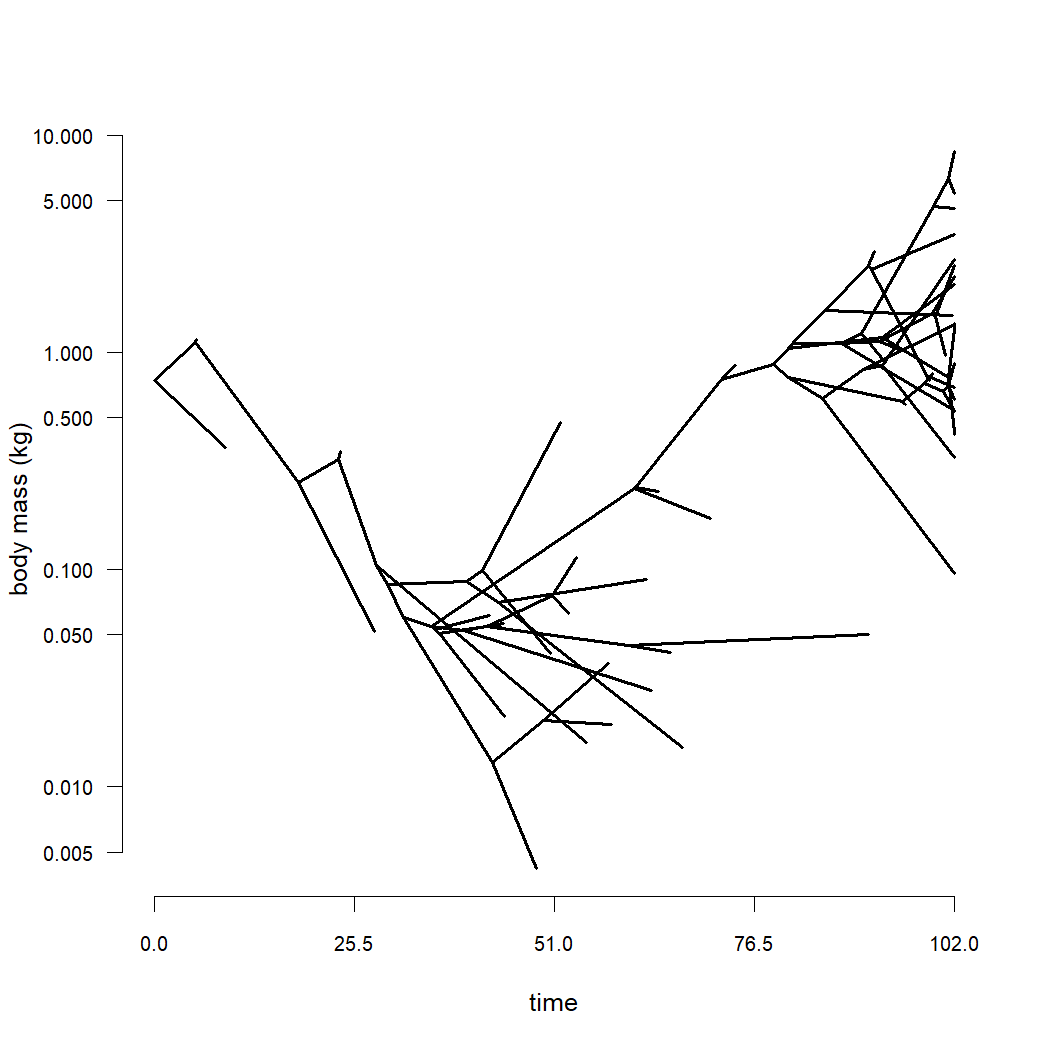OK, now what we'd like to do is draw a set of transects of slices through our tree, at each transect obtain ancestral state estimates for each of the edges bisected by the slice, and then compute the maximum value of these states.

I'll imagine that we want to compute these transects at intervals of 5 million years. Let's plot these transects on the tree.

``````## compute total tree height
h<-max(nodeHeights(tree))
## generate sampling points
interval<-5
dd<-seq(h,0,by=-interval)
## plot the sampling scheme
plotTree(tree,ftype="off",mar=c(5.1,4.1,1.1,1.1))
axis(1)
abline(v=dd,lty='dotted',col='grey')
``````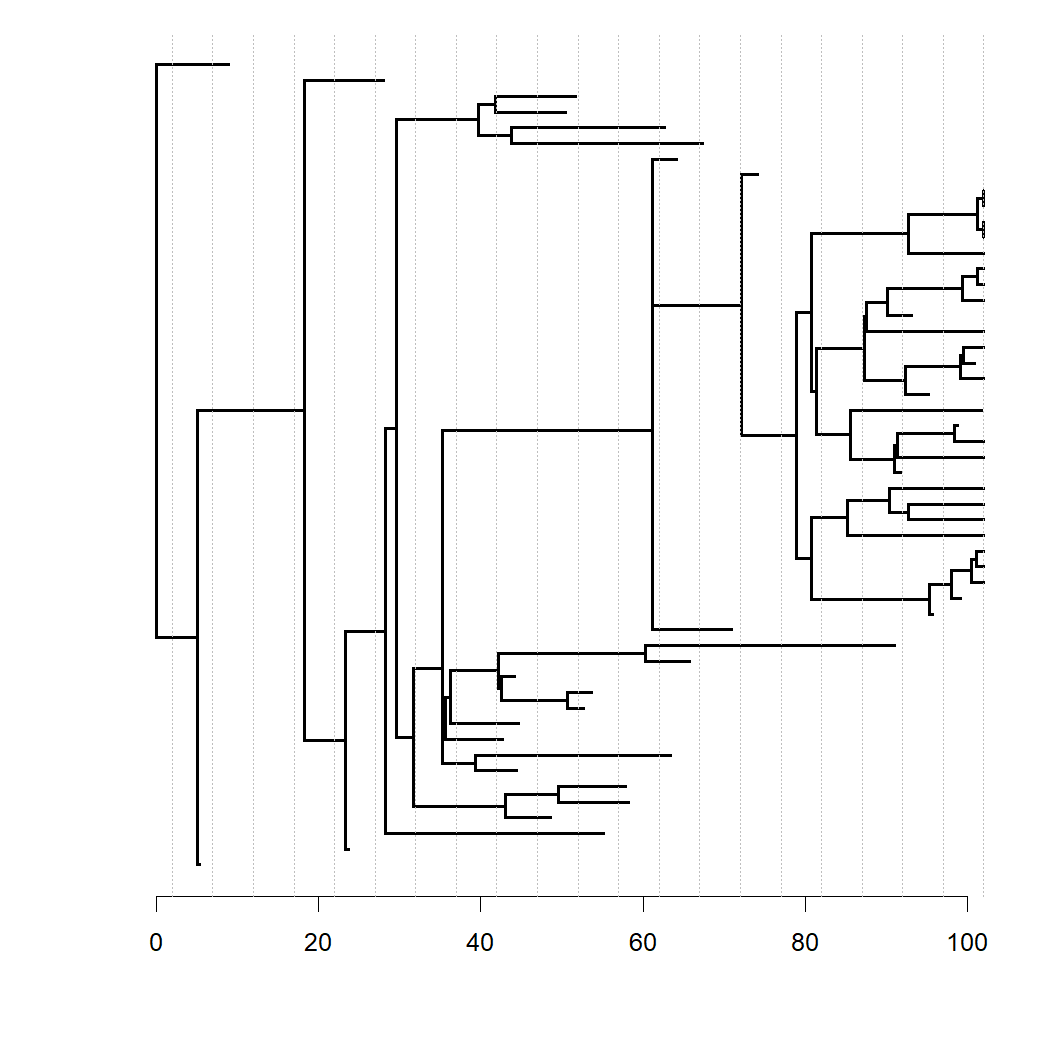Great.

So, our next step is to find the maximum value of our reconstructed (or observed, for present-day tips) of our phenotype at each time slice.

The way we'll do that is kind of interesting.

For each slice, we'll first use `make.era.map` to paint two different regimes on the tree. Then we'll using `map.to.singleton` to convert this regime shift into a set of singleton (i.e., unbranching) nodes. Lastly, we'll estimate ancestral states for all the nodes of the tree, pull out those corresponding to our time slice, and find the maximum.

``````## get maximum tip state
MAX<-max(x[getExtant(tree)])
## set tolerance
tol<-1e-8
## loop over internal time slices
for(i in 2:length(dd)){
obj<-map.to.singleton(make.era.map(tree,c(0,dd[i])))
a<-fastAnc(obj,x)
hh<-sapply(as.numeric(names(a)),nodeheight,tree=obj)
MAX[i]<-max(a[which(abs(hh-dd[i])<tol)])
}
``````

Now let's plot the values we've obtained. Here, I'll overlain our traitgram projection onto the plot of this value through time, just to make sure we obtained what we intended.

``````plot(dd,exp(MAX),type="b",bty="n",
ylim=exp(range(x)),pch=16,
log="y",xlab="time since the root",lwd=2,
ylab="maximum body mass (kg)",
las=1,cex.axis=0.8)
color=make.transparent("blue",0.1),lwd=4)
abline(v=dd,lty='dotted',col=make.transparent("grey",0.25))
``````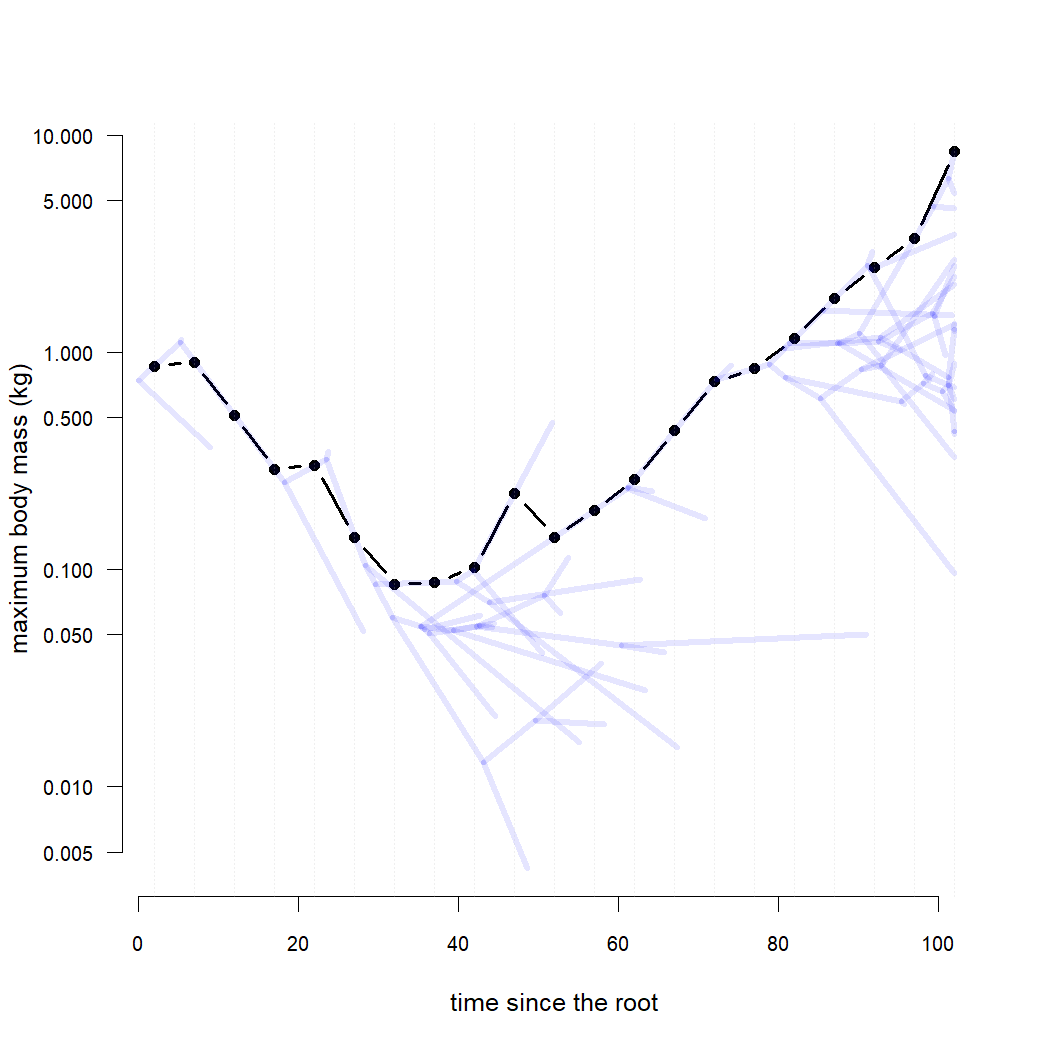That's pretty cool.

We can also do the same with the mean value across lineages. Note that for a Brownian model with only extant species this would really not be very interesting; however, if our tree includes fossil lineages than it might make sense. Let's try it.

``````MEAN<-mean(x[getExtant(tree)])
## set tolerance
tol<-1e-8
## loop over internal time slices
for(i in 2:length(dd)){
obj<-map.to.singleton(make.era.map(tree,c(0,dd[i])))
a<-fastAnc(obj,x)
hh<-sapply(as.numeric(names(a)),nodeheight,tree=obj)
MEAN[i]<-mean(a[which(abs(hh-dd[i])<tol)])
}
## plot with x-axis flipped to time before present
plot(h-dd,exp(MEAN),type="b",bty="n",xlim=c(h,0),
ylim=exp(range(x)),log="y",
xlab="time since the present",
ylab="mean body mass (kg)",
las=1,cex.axis=0.8)
``````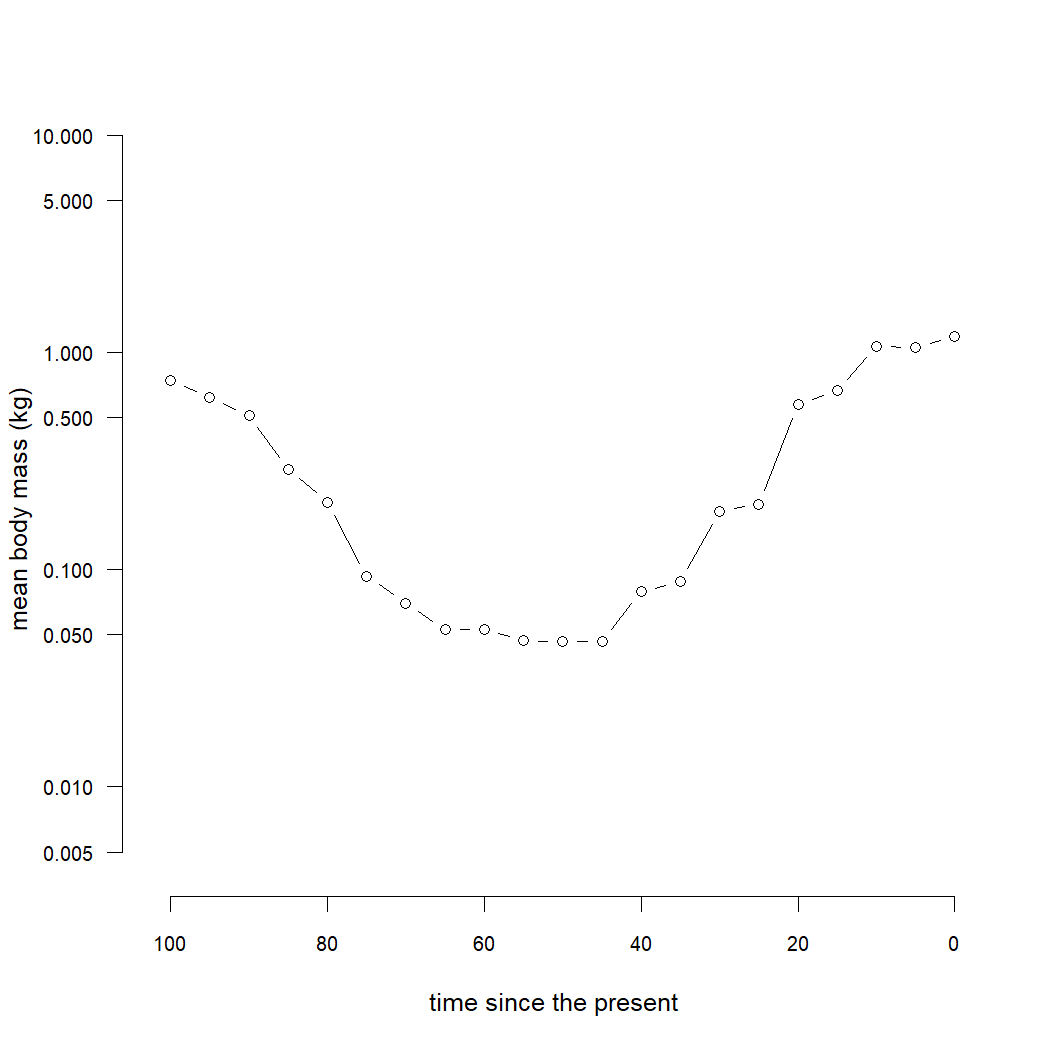Finally, let's consider data that may have evolved under a Brownian model with a trend.

Actually, this model is non-identifiable for an extant-only species tree; however, we can fit it to the case with a tree consisting of both extant & extinct lineages.

Let's simulate this & then try.

To simulate this I'll use `fastBM` with `mu` not equal to zero; and then to reconstruct ancestral states under a trend model I'll use `anc.trend`.

``````set.seed(9)
``````
``````y<-fastBM(tree,sig2=0.2,mu=0.1)
phenogram(tree,exp(c(anc.trend(tree,y)\$ace,y)),
ftype="off",log="y",las=1,cex.axis=0.8)
``````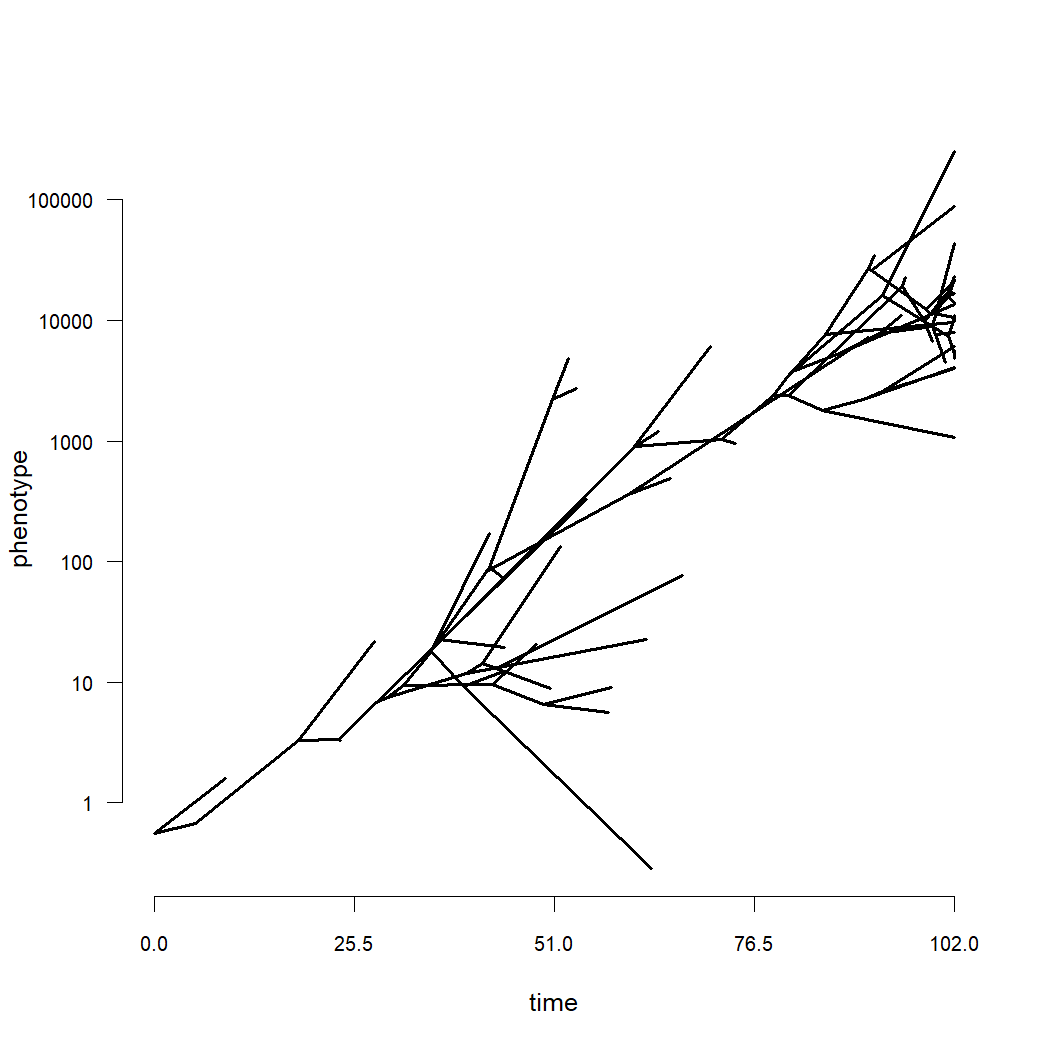``````MEAN<-mean(y[getExtant(tree)])
## set tolerance
tol<-1e-8
for(i in 2:length(dd)){
obj<-map.to.singleton(make.era.map(tree,c(0,dd[i])))
fit<-anc.trend(obj,y)
a<-fit\$ace
hh<-sapply(as.numeric(names(a)),nodeheight,tree=obj)
MEAN[i]<-mean(a[which(abs(hh-dd[i])<tol)])
}
plot(h-dd,exp(MEAN),type="b",bty="n",xlim=c(h,0),
log="y",xlab="time since the present",
ylab="mean body mass (kg)",
las=1,cex.axis=0.8)
``````